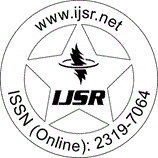International Journal of Science and Research (IJSR)
Call for Papers | Fully Refereed | Open Access | Double Blind Peer Reviewed

Research Paper | Mathematics | India | Volume 4 Issue 11, November 2015

# Matter Symmetries of Linet-Tian Metric in General Theory of Relativity

R. S. Rane | S. S. Dabhane

Abstract: In this paper, we have discussed the symmetries of the energy-momentum tensor (T_ab) for the Linet-Tian metric. We have obtained different constraint equations on energy-momentum tensor (T_ab). If we solve these constraint equations, we obtain new exact solutions of Einstein field equations. We have solved matter symmetries (collineations) equations for the four main cases by taking one, two, three and four non-zero components of the energy-momentum tensor (T_ab). We have investigated the degenerate case i. e. det (T_ab) =0 for one, two and three non-zero components energy-momentum tensor (T_ab) and non-degenerate case i. e. det (T_ab) -0 for all four components of energy-momentum tensor (T_ab) are non-zero. It is observed that, this space-time have infinite number of matter symmetries (collineations) in degenerate case and have finite number of matter symmetries in non-degenerate case. It is also observed that this space-time gives seven independent matter symmetries in which three are linearly independent Killing vectors and the remaining are dependent.

Keywords: Linet-Tian metric, matter symmetries collineations, energy-momentum tensor

Edition: Volume 4 Issue 11, November 2015,

Pages: 2019 - 2023